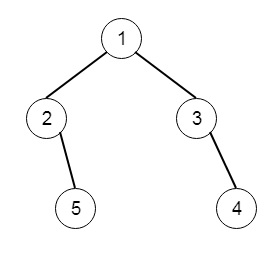# Binary Tree Right Side View in C++

Suppose we have a binary tree, if we see the tree from right side, then we can see some elements of it. we have to display those elements. So if the tree is like −To solve this, we will follow these steps −

• We will create one helping method for dfs. This will take tree_node, an array to hold answers, and level. The level is initially 0. The dfs will work like below −
• if node is null, then return
• if level = length of the answer array, then insert value of node into the ans array
• dfs(right of the node, ans, level + 1)
• dfs(left of the node, ans, level + 1)
• From the main function call the dfs() using the root of the tree and one blank array, the level is initially 0, so this will be dfs(root, ans)

Let us see the following implementation to get better understanding −

## Example

Live Demo

#include <bits/stdc++.h>
using namespace std;
void print_vector(vector<int> v){
cout << "[";
for(int i = 0; i<v.size(); i++){
cout << v[i] << ", ";
}
cout << "]"<<endl;
}
class TreeNode{
public:
int val;
TreeNode *left, *right;
TreeNode(int data){
val = data;
left = right = NULL;
}
};
void insert(TreeNode **root, int val){
queue<TreeNode*> q;
q.push(*root);
while(q.size()){
TreeNode *temp = q.front();
q.pop();
if(!temp->left){
if(val != NULL)
temp->left = new TreeNode(val);
else
temp->left = new TreeNode(0);
return;
} else {
q.push(temp->left);
}
if(!temp->right){
if(val != NULL)
temp->right = new TreeNode(val);
else
temp->right = new TreeNode(0);
return;
} else {
q.push(temp->right);
}
}
}
TreeNode *make_tree(vector<int> v){
TreeNode *root = new TreeNode(v);
for(int i = 1; i<v.size(); i++){
insert(&root, v[i]);
}
return root;
}
class Solution {
public:
void dfs(TreeNode* node, vector <int>& ans, int level = 0){
if(!node) return;
if(level == ans.size())ans.push_back(node->val);
dfs(node->right, ans, level + 1);
dfs(node->left, ans, level + 1);
}
vector<int> rightSideView(TreeNode* root) {
vector <int> ans;
dfs(root, ans);
return ans;
}
};
main(){
vector<int> v = {1,2,3,NULL,5,NULL,4};
TreeNode *root = make_tree(v);
Solution ob;
print_vector(ob.rightSideView(root));
}

## Input

[1,2,3,null,5,null,4]

## Output

[1, 3, 4, ]